# Purpose

vitesse is a software for computing the extremal articular velocties of a parallel manipulator whose end-effector should be able to reach a cartesian/ angular velocity for any position in a given workspace

# Availability

The source of this software is freely available via anonymous fpt (download here).

This software is provided "as is" without warranty of any kind. In no event shall INRIA be liable for any loss of profits, loss of business, loss of use or data, interruption of business, or for indirect, special, incidental, or consequential damages of any kind, arising from any error in this software.

# Implementation features

Window system: none
Type: source file
Size of binary: 0.37 Mo
Output: numerical result

# User's manual

User's manual of vitesse
Version 0.2
7/27/1997

# Introduction

vitesse is a software enabling to compute the extremal (minimal and maximal) articular velocities of parallel robot of the Gough platform type which are necessary for the end-effector to perform a given cartesian/articular velocity when it is moving in a given workspace with a constant orientation. The extremal articular velocities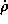are computed with a guaranteed errorso that if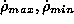are the real extremal velocities over the workspace and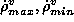are the results of the program, then the following relation hold: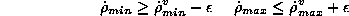This is an implementation of the algorithm which will be described in a paper submitted to the 1998 IEEE Conference on Robotics and Automation.

# Using the program

The program is invoked with one argument which is a file (called a configuration file) containing all the data. An example of the file is the following:

```
robot_file new.car
orientation -60 -60 -60
velocity -10 0 -10 -10 0 10
speed_accuracy 0.05
region -10 10 -10 15 -20 20```
The sentence robot_file new.car indicates that we are studying a robot whose geometry is defined in the file new.car. In this file you should indicate line by line the 3 coordinates of the 6 centers of the joints located on the base, then coordinates of the 6 centers of the joints located on the moving platform. Optionally you may indicate on the next line the 6 maximal values of the leg lengths and on the next line their 6 minimal values. The sentence orientation -60 -60 -60 indicates the orientation of the moving platform over the desired workspace. This the Euler angle of the platform in degree (here the Euler angles are defined by the rotation angle around the z axis, then the rotation angle around the new x axis, then the rotation angle around the new z axis).

The sentence velocity -10 0 -10 -10 0 10 indicates the cartesian/angular velocities for which we want to determine the extremal articular velocities. The angular velocities are expressed in degree/s.

The sentence speed_accuracy 0.05 indicates the accuracywith which the extremal velocities are to be computed.

The sentence region -10 10 -10 15 -20 20 indicate that the workspace of the end-effector is defined as a box with x in [-10,10], y in [-10,15], z in [-20,20]. This sentence can be changed to:

```
region_polygon my-zone```
where my-zone is a file which contain horizontal polygonal cross-sections of the desired workspace at various height. Such a file is for example:
```
z 50
-10 -10
-10 10
10 10
10 -10
z 55
-5 -5
-5 5
5 5
5 -5
z 60
-10 -10
-10 10
10 10
10 -10```
Here we have given 3 cross-sections at z=50,55,60. Each cross-section is defined by pairs of coordinates which indicate the vertices of the polygon. Note that it is necessary that the cross-sections have exactly the same number of vertices. The volume between 2 cross-section is obtained simply by a linear interpolation.

Instead of using region and region_polygon you may also use the keyword workspace. In that case the workspace is defined as all the positions of the platform such that the leg lengths limits specified in the robot file are respected. You may also use special version of this keyword: workspace>0 and workspace<0 followed by a number. In that case the position that will be considered will have a z value which is larger (lower) or equal to the specified number. After having defined you data in a file you just run the program and it will print the result. If you have defined your workspace to be a box you may verify the result with a discrete method (which is computer intensive).

# Computation time

## Box workspace

If the angular velocity of the end-effector is equal to 0 the results are exact and the computation time is low (about 10-20ms on a SUN Ultra 1 workstation). Otherwise the computation time is clearly dependent upon the accuracy. For the configuration file presented here as an example the computation time as a function of the accuracyis presented in the figure 1: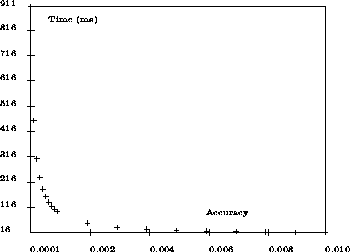Figure 1:   Computation time as a function of the precision on the value of the extremal velocities

You may notice that even with a very high accuracy the computation time is still low.

## Cross-sections workspace

In fact for computing the articular velocities in that case we use the box algorithm: between two cross-sections the volume is split into boxes until we are sure that a further splitting will not improve the accuracy of the result. Consequently the computation time will heavily depend upon the accuracy. For the example presented in this manual the following table indicates the computation time as function of the accuracy: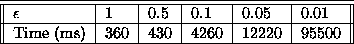Table 1:   Computation time for a complex workspace as function of the desired accuracyon the articular velocities

But if the angular velocity is equal to 0 a faster algorithm is used which lead to an exact result and to a computation time of about 17ms on a SUN Ultra 1 workstation.

For the full workspace the computation time vary from 1 to 2 mn according to the accuracy.

# Useful procedures

In the file you may find some particular procedures which may be of interest in some special cases.

## For a segment trajectory

If you want to check the extremal articular velocities when your end-effector is moving on a segment you may use

```
max_min_vitesse_art_num_segment_fast(num,start,goal,angle,speed,
speed_min,speed_max,lamm)```
where num indicates the leg number, start, goal contain the three coordinates of the extremities of the segment trajectory, angle defines the orientation of the platform by the three Euler angles in radian, speed is cartesian/angular velocity of the end-effector, speed_min, speed_max are the extremal articular velocities and lamm is a 6x2 array which give the location of the end-effector for which the extremal articular velocities are obtained. If minimal, maximal are these locations we have
```
minimal= start+lamm[num]*(goal-start)
maximal= start+lamm[num]*(goal-start)```
If you have defined the minimal and maximal leg lengths you can also check the articular velocities for a segment without worrying about the workspace you may use:
```
max_vitesse_articulaire_segment(start,goal,angle,speed,speed_ro,lam,nb,lamm)```
This procedure check the velocities for all the legs and put the result in the 6x2 array speed_ro. But the velocities are only computed for the parts of the segment trajectory which respect the constraint on the leg lengths and indicates in the 20x2 array lam the nb intervals which define the invalid portion of the segment trajectory (i.e. the part of the segment which are outside the workspace of the robot). An invalid portion AB is defined by:
```
A= start+lam[i]*(goal-start)
B= start+lam[i]*(goal-start)```

All these procedures lead to an exact result.

## For a horizontal rectangle

If you want to check the extremal articular velocities defined by an horizontal rectangle you may use:

```
Max_speed_region(Z,angle,speed,speed_ro,borne)```
where Z is the height of the rectangle, the 6x2 array speed_ro is the result, borne is an array which contain in this order: the minimal x, the maximal x, the minimal y, the maximal y. The results are exact.

## For the full workspace

Use:

```
Check_speed_bi_workspace(angle,speed,dro_max,pm,PM)```
dro_max is double ro_max and will contain the minimum and maximum of the articular velocities. For example dro_max represent the minimal articular velocity of leg 1 (the leg number start at 0) while dro_max is the maximal articular velocity. pm, pM are double pm, pM and will contain the location of the position where the minimal and maximal articular velocity occur. The variable type_workspace should be put to 0 is the full workspace is to be checked while a positive (negative) value will indicate that you want to check the part of the workspace such that z is greater (lower) than the given value.

# History

Version 0.1: 21/7/1996
Version 0.2: 27/7/1997

Jean-Pierre Merlet@Mon Jul 28 16:37:01 MET DST 1997